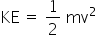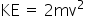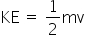Physics
Easy

Question

# Identify the correct formula for calculating kinetic energy.

##KE = 2mvHint:

## The correct answer is:### Kinetic energy is directly proportional to the mass of the object and to the square of its velocity, that is, K.E.=1/2 mv².If the mass has units of kilograms and the velocity of metres per second, the kinetic energy has units of kilograms metres Squared per second squared.#### With Turito Foundation.#### Get an Expert Advice From Turito.